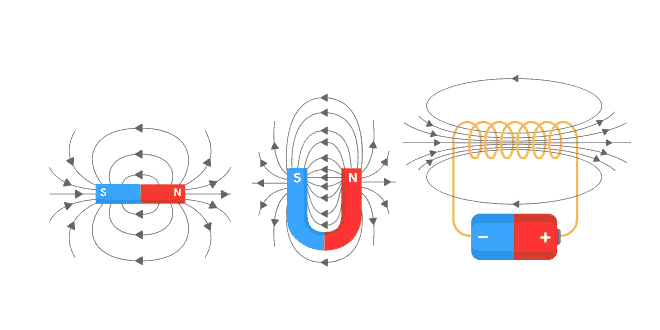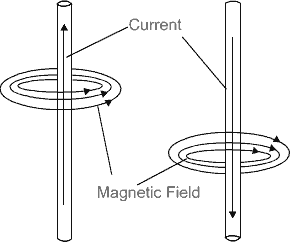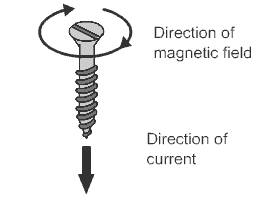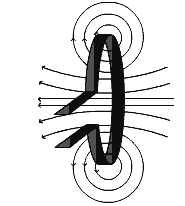Monday , March 30 2020# Magnetic Effects of Electric Current Quiz: 10 Science

## NCERT 10th Class (CBSE) Science: Magnetic Effects of Electric Current Quiz

#### 23 Multiple Choice Questions related to NCERT 10th Class (CBSE) Science (Physics) Magnetic Effects of Electric Current: Quiz

• Every current carrying conductor has a magnetic field around it.
• If field lines of a magnetic field cross each other, it would mean that at the point of intersection, the compass needle would point towards two directions, which is not possible.
• A cylindrical coil of many tightly would turns of insulated wires with generally diameter of the coil smaller than its length is called a solenoid.
• It depends upon: (i) the current through the solenoid, (ii) the number of turns in the solenoid, and (iii) nature of the core on which the solenoid is would.

## Properties of magnet:

• A free suspended magnet always point towards north and south direction.
• The pole of a magnet which points toward north direction is called north pole or north seeking.
• The pole of a magnet which points toward south direction is called south pole or south seeking.
• Like poles of magnets repel each other while unlike poles of magnets attract each other.

Similar to other effects; electric current also produces magnetic effect. The magnetic effect of electric current is known as electromagnetic effect.

It is observed that when a compass is brought near a current carrying conductor the needle of compass gets deflected because of flow of electricity. This shows that electric current produces a magnetic effect.

## Magnetic field and Field Lines

The influence of force surrounding a magnet is called magnetic field. In the magnetic field, the force exerted by a magnet can be detected using a compass or any other magnet.

The imaginary lines of magnetic field around a magnet are called field line or field line of magnet. When iron fillings are allowed to settle around a bar magnet, they get arranged in a pattern which mimics the magnetic field lines. Field line of a magnet can also be detected using a compass. Magnetic field is a vector quantity, i.e. it has both direction and magnitude.

Direction of Field Line: Outside the magnet, the direction of magnetic field line is taken from north pole to South Pole. Inside the magnet, the direction of magnetic field line is taken from south pole to north pole.

Strength of magnetic field: The closeness of field lines shows the relative strength of magnetic field, i.e. closer lines show stronger magnetic field and vice-versa. Crowded field lines near the poles of magnet show more strength.

## Magnetic field Due to a Current Carrying Conductor:

#### Magnetic field due to current through a straight conductor:

A current carrying straight conductor has magnetic field in the form of concentric circles; around it. Magnetic field of current carrying straight conductor can be shown by magnetic field lines.

The direction of magnetic field through a current carrying conductor depends upon the direction of flow of electric current. The direction of magnetic field gets reversed in case of a change in the direction of electric current.

Let a current carrying conductor be suspended vertically and the electric current is flowing from south to north. In this case, the direction of magnetic field will be anticlockwise. If the current is flowing from north to south, the direction of magnetic field will be clockwise.

## Right Hand Thumb Rule:The direction of magnetic field; in relation to direction of electric current through a straight conductor can be depicted by using the Right Hand Thumb Rule. It is also known as Maxwell’s Corkscrew Rule.If a current carrying conductor is held by right hand; keeping the thumb straight and if the direction of electric current is in the direction of thumb, then the direction of wrapping of other fingers will show the direction of magnetic field.As per Maxwell’s corkscrew rule, if the direction of forward movement of screw shows the direction of current, then the direction of rotation of screw shows the direction of magnetic field.

Properties of Magnetic Field:

• The magnitude; of magnetic field increases with increase in electric current and decreases with decrease in electric current.
• The magnitude of magnetic field; produced by electric current; decreases with increase in distance and vice-versa. The size of concentric circles of magnetic field lines increases with distance from the conductor, which shows that magnetic field decreases with distance.
• Magnetic field lines are always parallel to each other.
• No two field lines cross each other.

## Magnetic field due to current through a circular loop:In case of a circular current carrying conductor, the magnetic field is produced in the same manner as it is in case of a straight current carrying conductor.

In case of a circular current carrying conductor, the magnetic field lines would be in the form of concentric circles around every part of the periphery of the conductor. Since, magnetic field lines tend to remain closer when near the conductor, so the magnetic field would be stronger near the periphery of the loop. On the other hand, the magnetic field lines would be distant from each other when we move towards the centre of the current carrying loop. Finally; at the centre, the arcs of big circles would appear as a straight lines.

The direction of magnetic field can be identified using Right Hand Thumb’s Rule. Let us assume that the current is moving in anti-clockwise direction in the loop. In that case, the magnetic field would be in clockwise direction; at the top of the loop. Moreover, it would be in anticlockwise direction at the bottom of the loop.

Clock Face Rule: A current carrying loop works like a disc magnet. The polarity of this magnet can be easily understood with the help of clock face rule. If the current is flowing in anti-clockwise direction, then the face of the loop shows north pole. On the other hand, if the current is flowing in clockwise direction, then the face of the loop shows south pole.

Magnetic field and number of turns of coil:Magnitude of magnetic field gets summed up with increase in the number of turns of coil. If there are ‘n’ turns of coil, magnitude of magnetic field will be ‘n’ times of magnetic field in case of a single turn of coil.

### Magnetic Field due to a current in a Solenoid:

Solenoid is the coil with many circular turns of insulated copper wire wrapped closely in the shape of cylinder.

A current carrying solenoid produces similar pattern of magnetic field as a bar magnet. One end of solenoid behaves as the north pole and another end behaves as the south pole. Magnetic field lines are parallel inside the solenoid; similar to a bar magnet; which shows that magnetic field is same at all points inside the solenoid.

By producing a strong magnetic field inside the solenoid, magnetic materials can be magnetized. Magnet formed by producing magnetic field inside a solenoid is called electromagnet.

## लखनवी अंदाज़ 10th Class Hindi क्षितिज भाग 2 Chapter 12

लखनवी अंदाज़ 10th Class Hindi क्षितिज भाग 2 (गद्य खंड) Chapter 12 प्रश्न: लेखक को नवाब …